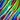# Verify record in HTML table

Though not very often encountered, this again is an interesting problem to solve in automation testing. It’s quite obvious to come across table validation as an automation tester and there are quite a lot of options to do that. The most common verifications include

1. Verifying a unique identifier in a column
2. Verifying a specific text (cell) in a table based on a unique identifier
3. Verifying a specific row (multiple values) in a table based on multiple identifiers (whether row exists)

Hosted table for testing here and HTML Table source here

The basic solution, as discussed here, solves 80% of all the listed problems. However, that only works when the table has a unique identifier. The solution for points 1 and 2 are already part of the previous post. So, let’s see how to solve the next complex problem # 3

This is again not a complex one to understand, however quite complex to validate with just xpath. There is still an option to validate this with some basic xpath’s and handling the validation part in the script/code but we are not gonna see that here for the same reason mentioned in my previous post. And it’s way too costly in this case.

To make things clear, the problem that we intend to solve here is

We have a table with multiple columns and rows; and our task is to validate whether a record exist with 3 or more values in a specific order in a row, or in other words, under specific headers. And we intend to solve this using only xpath i.e., without iteration in the code.

Let’s take on one of the complex records to find in the table. In our example, let’s plan to find the value CodePen under the header Sites and 2002 under the header Views and 4135 under the header Clicks

For our initial test, let’s first assume that we already know the index of the header column for each of these values that we need to verify in which case, the xpath would be something like

//tr[td[.='CodePen' and position()=1] and td[.='2002' and position()=2] and td[.='4135' and position()=3]]

As you can see, the position() keyword comes in handy in this case. So, the actual code to validate these values would be

String expectedValue1 = "CodePen";
String expectedValue2 = "2002";
String expectedValue3 = "4135";

String derivedXpath = "//tr[td[.='" + expectedValue1 + "' and position()=1] and td[.='" + expectedValue2 + "' and position()=2] and td[.='" + expectedValue3 + "' and position()=3]]";

boolean isElementPresent = driver.findElements(By.xpath(derivedXpath)).size() > 0;

if (isElementPresent) {
// Do something after test step is passed
} else {
// Do something after test step is failed
}

Moving on to the next part where you do not know the position of each of the headers from which you need to validate the value. If you have already read my previous post on verifying text in HTML table, you might probably have guessed that we can use the count() operator to find the index! And guess what, that is correct 🙂

However, this time instead of verifying the existence of the header, we will be using a different approach. We will still use the count() operator, but in conjunction with number() and boolean() operators to validate whether the header exists. The xpath to get the position of a particular header would be

count(//th[.='Clicks']/preceding-sibling::th)+number(boolean(//th[.='Clicks']))

The above xpath will get the count of preceding siblings with tag name ‘th’ and then adds the boolean value for the existence of the object. Basically, the number() operator returns 1 (true)/0 (false) based on the input passed into it and the boolean() operator returns true/false based on the object existence.

In simple terms

• boolean(Object) – true // Object is any matching tag from the given xpath
• boolean(null) – false // null is no matching tag from the given xpath
• number(true) – 1
• number(false) – 0

The new xpath would be

//tr[td[.='CodePen' and position()=count(//th[.='Sites']/preceding-sibling::th)+number(boolean(//th[.='Sites']))] and td[.='2002' and position()=count(//th[.='Views']/preceding-sibling::th)+number(boolean(//th[.='Views']))] and td[.='4135' and position()=count(//th[.='Clicks']/preceding-sibling::th)+number(boolean(//th[.='Clicks']))]]

Hope you get the idea. If so, all you gotta do is to parameterize the position values in the above code. The new code would now be

String expectedHeader1 = "Sites";

String expectedValue1 = "CodePen";
String expectedValue2 = "2002";
String expectedValue3 = "4135";

String derivedXpath = "//tr[td[.='" + expectedValue1 + "' and position()=count(//th[.='" + expectedHeader1 + "']/preceding-sibling::th)+number(boolean(//th[.='" + expectedHeader1 + "']))] and td[.='" + expectedValue2 + "' and position()=count(//th[.='" + expectedHeader2 + "']/preceding-sibling::th)+number(boolean(//th[.='" + expectedHeader2 + "']))] and td[.='" + expectedValue3 + "' and position()=count(//th[.='" + expectedHeader3 + "']/preceding-sibling::th)+number(boolean(//th[.='" + expectedHeader3 + "']))]]";

boolean isElementPresent = driver.findElements(By.xpath(derivedXpath)).size() > 0;

if (isElementPresent) {
// Do something after test step is passed
} else {
// Do something after test step is failed
}

It’s a quite complex xpath to derive when it comes to validating dynamic values with multiple headers and values, but considering the amount of time this xpath saves in execution, I would say “worth it!”